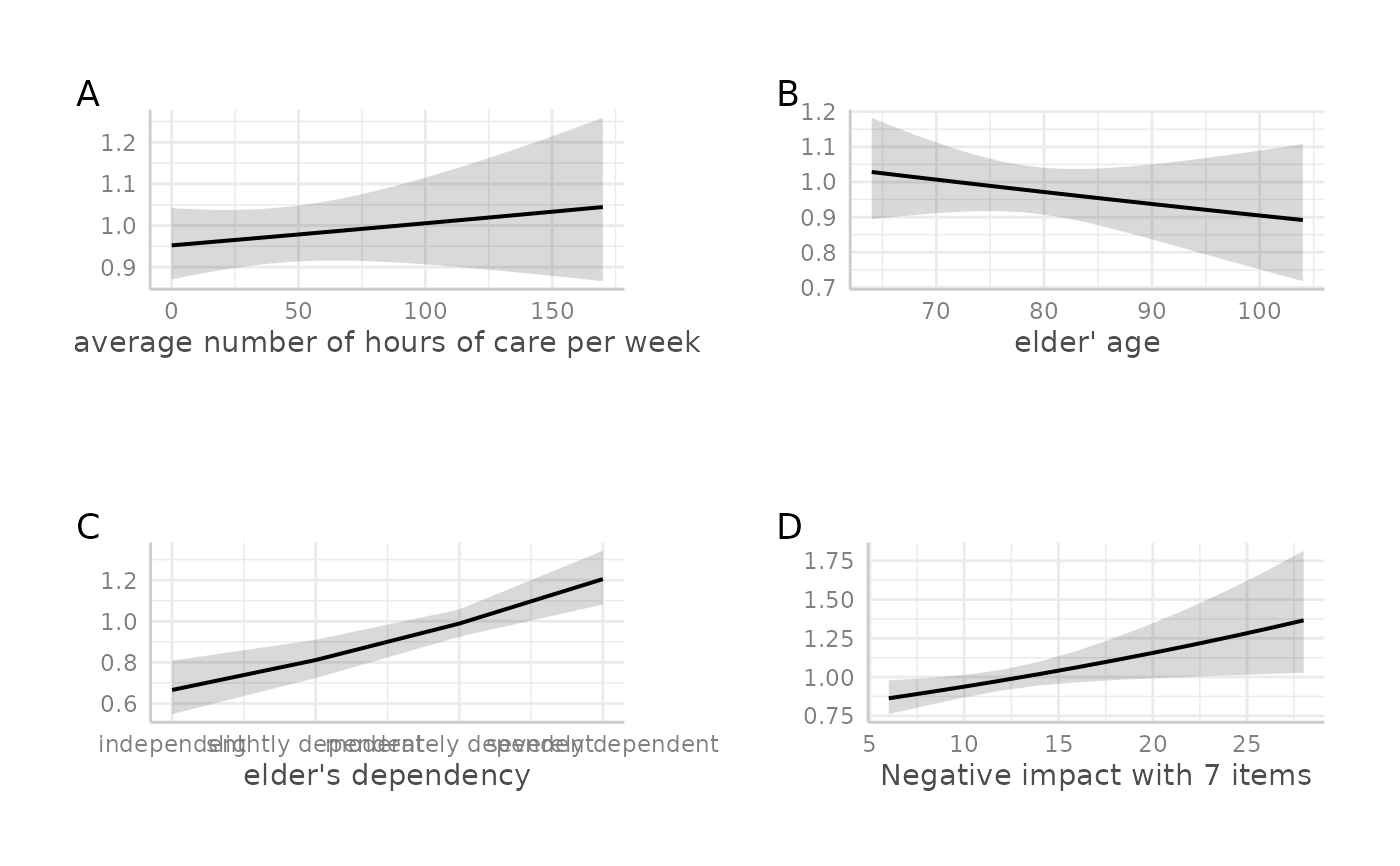Plot multiple ggplot-objects as a grid-arranged single plot.

## Usage

plot_grid(x, margin = c(1, 1, 1, 1), tags = NULL)

## Arguments

x

A list of ggplot-objects. See 'Details'.

margin

A numeric vector of length 4, indicating the top, right, bottom and left margin for each plot, in centimetres.

tags

Add tags to your subfigures. Can be TRUE (letter tags) or character vector containing tags labels.

## Value

An object of class gtable.

## Details

This function takes a list of ggplot-objects as argument. Plotting functions of this package that produce multiple plot objects (e.g., when there is an argument facet.grid) usually return multiple plots as list (the return value is named plot.list). To arrange these plots as grid as a single plot, use plot_grid.

## Examples

if (require("dplyr") && require("gridExtra")) {
library(ggeffects)
data(efc)

# fit model
fit <- glm(
tot_sc_e ~ c12hour + e17age + e42dep + neg_c_7,
data = efc,
family = poisson
)

# plot marginal effects for each predictor, each as single plot
p1 <- ggpredict(fit, "c12hour") %>%
plot(show.y.title = FALSE, show.title = FALSE)
p2 <- ggpredict(fit, "e17age") %>%
plot(show.y.title = FALSE, show.title = FALSE)
p3 <- ggpredict(fit, "e42dep") %>%
plot(show.y.title = FALSE, show.title = FALSE)
p4 <- ggpredict(fit, "neg_c_7") %>%
plot(show.y.title = FALSE, show.title = FALSE)

# plot grid
plot_grid(list(p1, p2, p3, p4))

# plot grid
plot_grid(list(p1, p2, p3, p4), tags = TRUE)
}
#> Warning: Not enough tags labels in list. Using letters instead.